# A Parameter Recovery with Reduction from P-values

## A.1 Individual Bias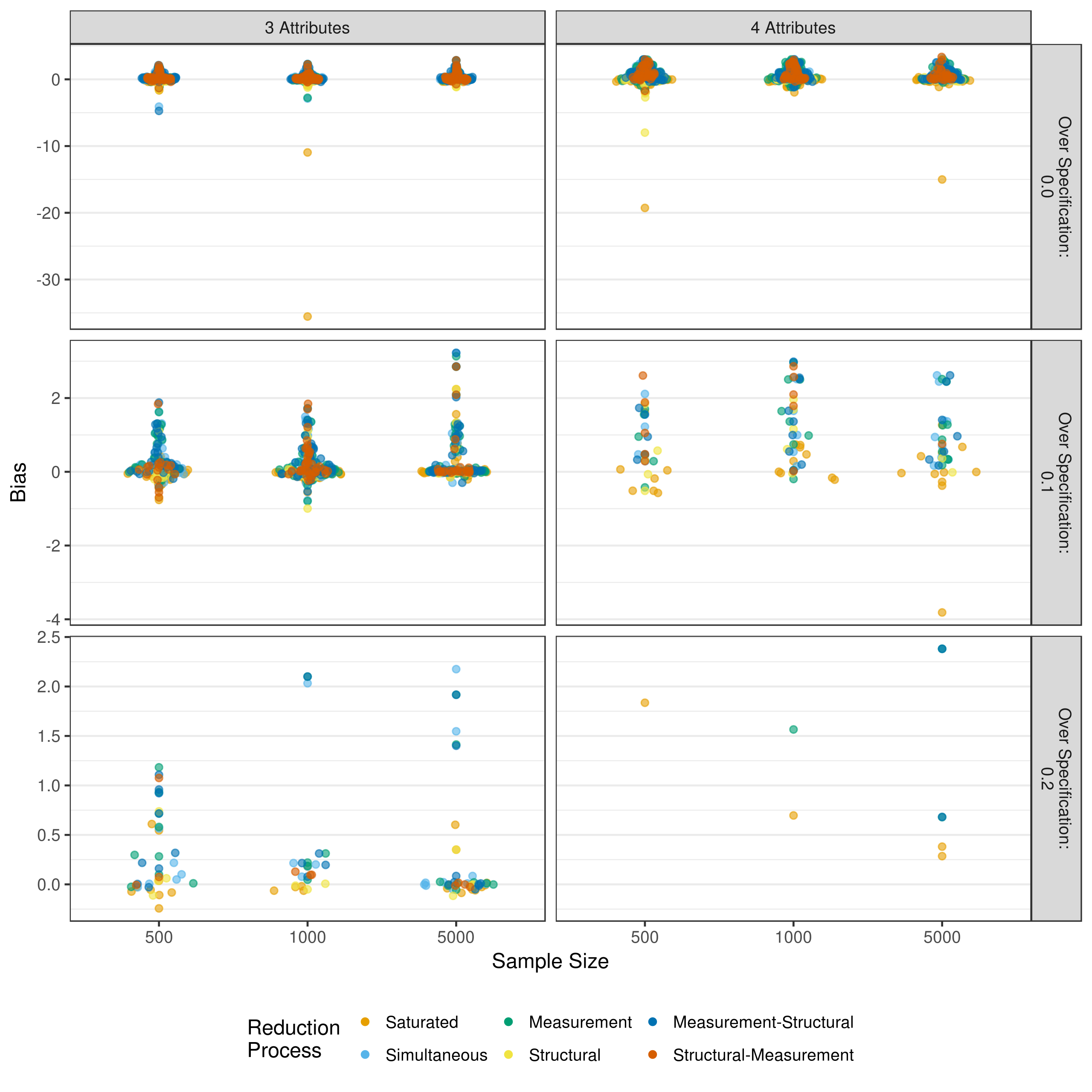Figure A.1: Bias in measurement model intercept estimates when reducing using p-values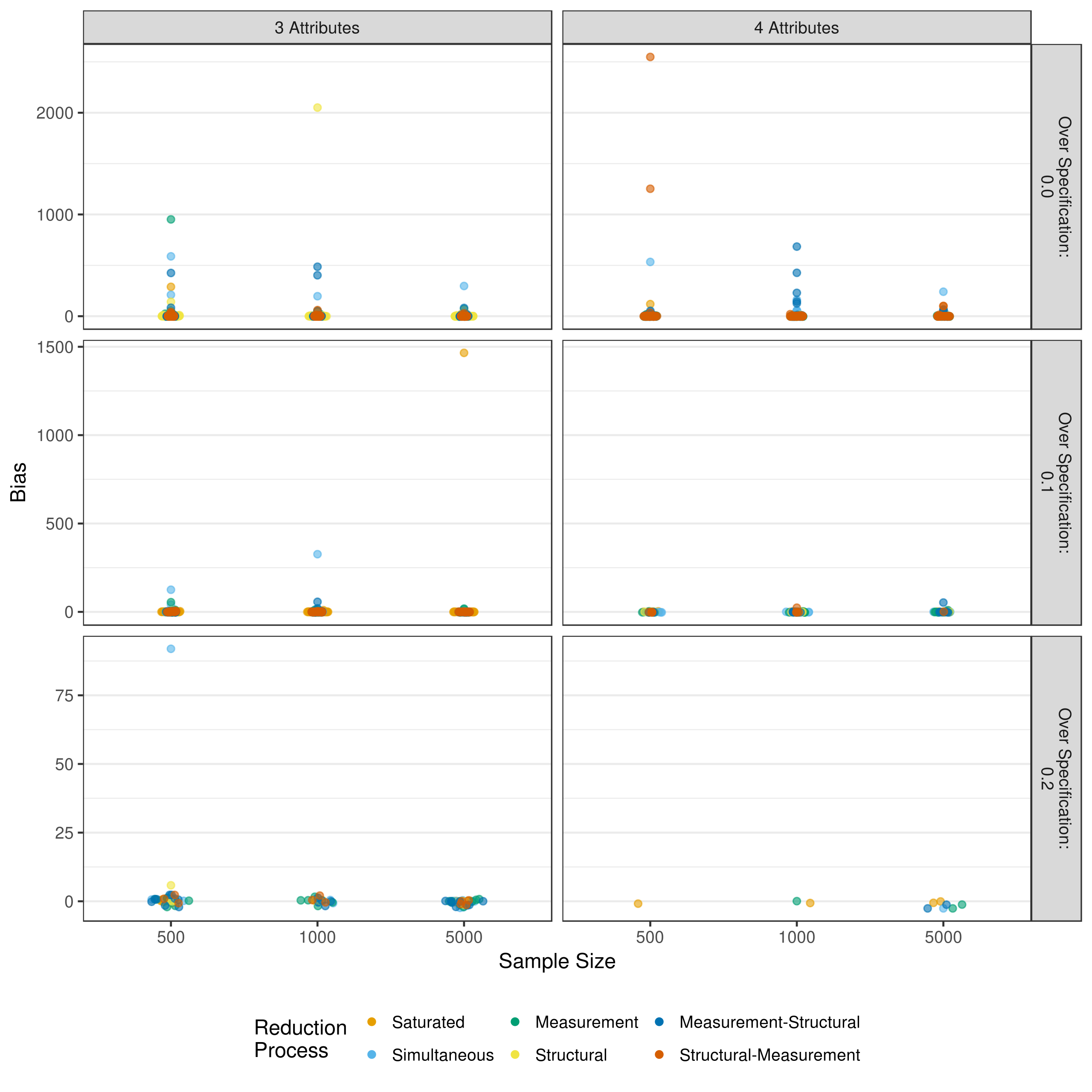Figure A.2: Bias in measurement model main effect estimates when reducing using p-values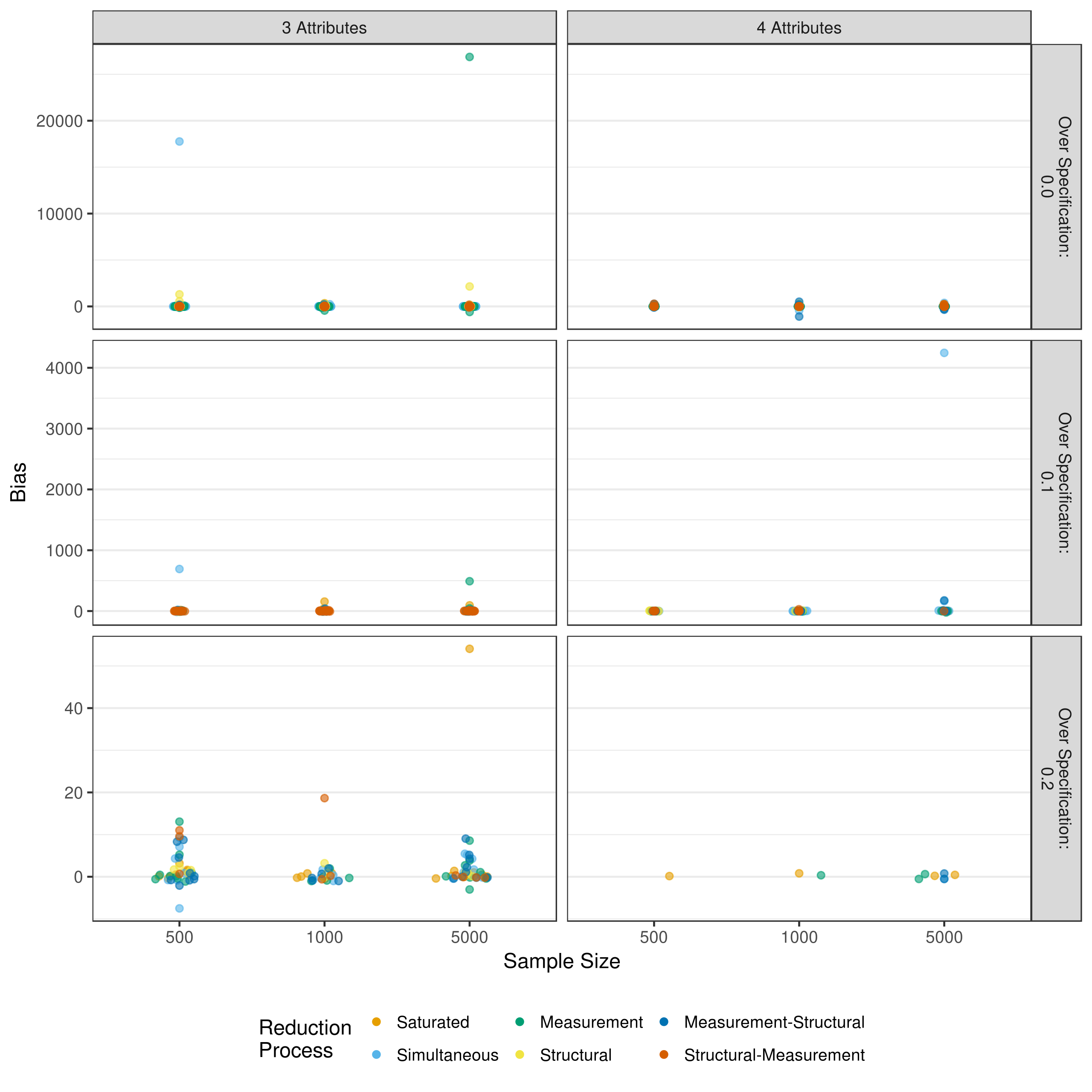Figure A.3: Bias in measurement model 2-way interaction estimates when reducing using p-values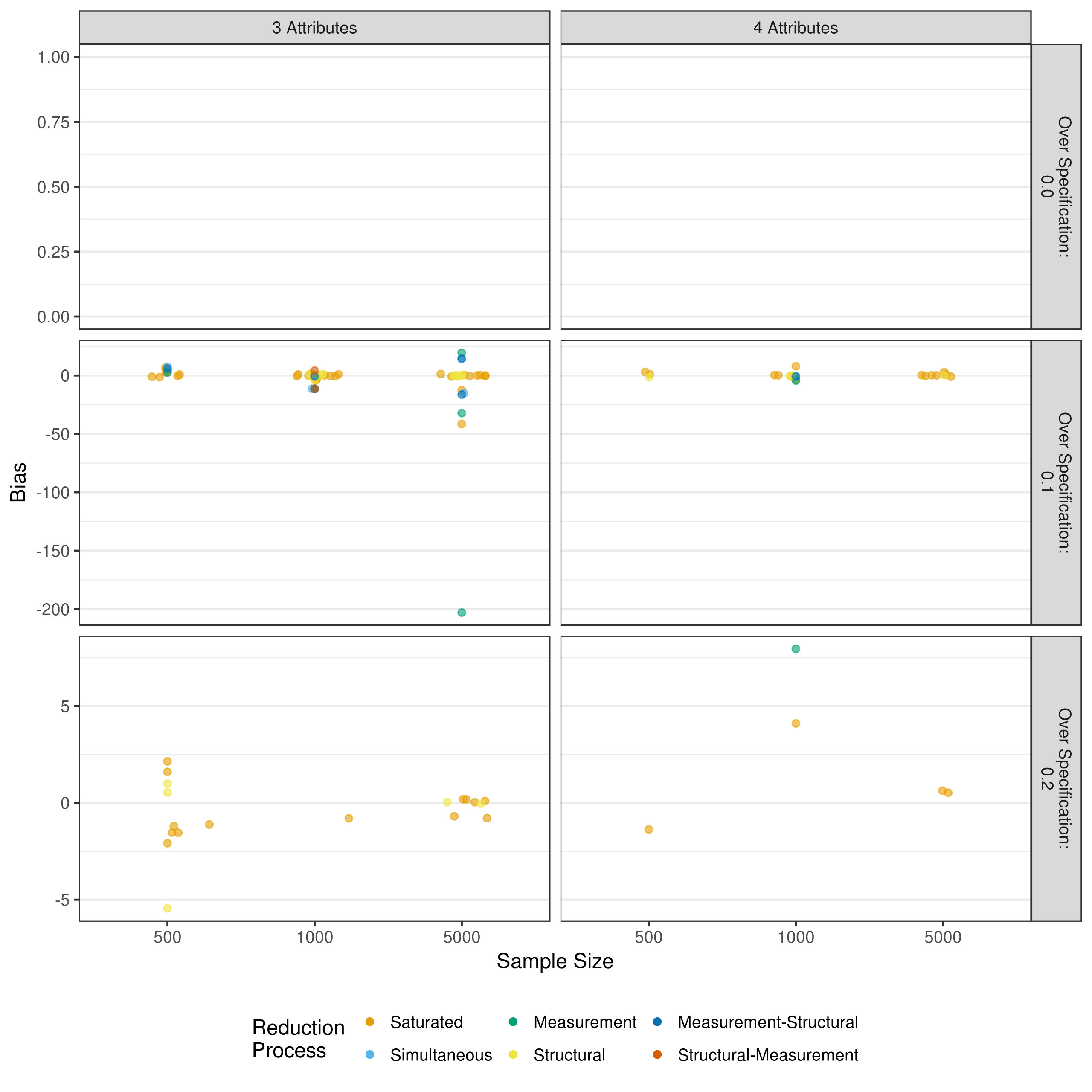Figure A.4: Bias in measurement model 3-way interaction estimates when reducing using p-values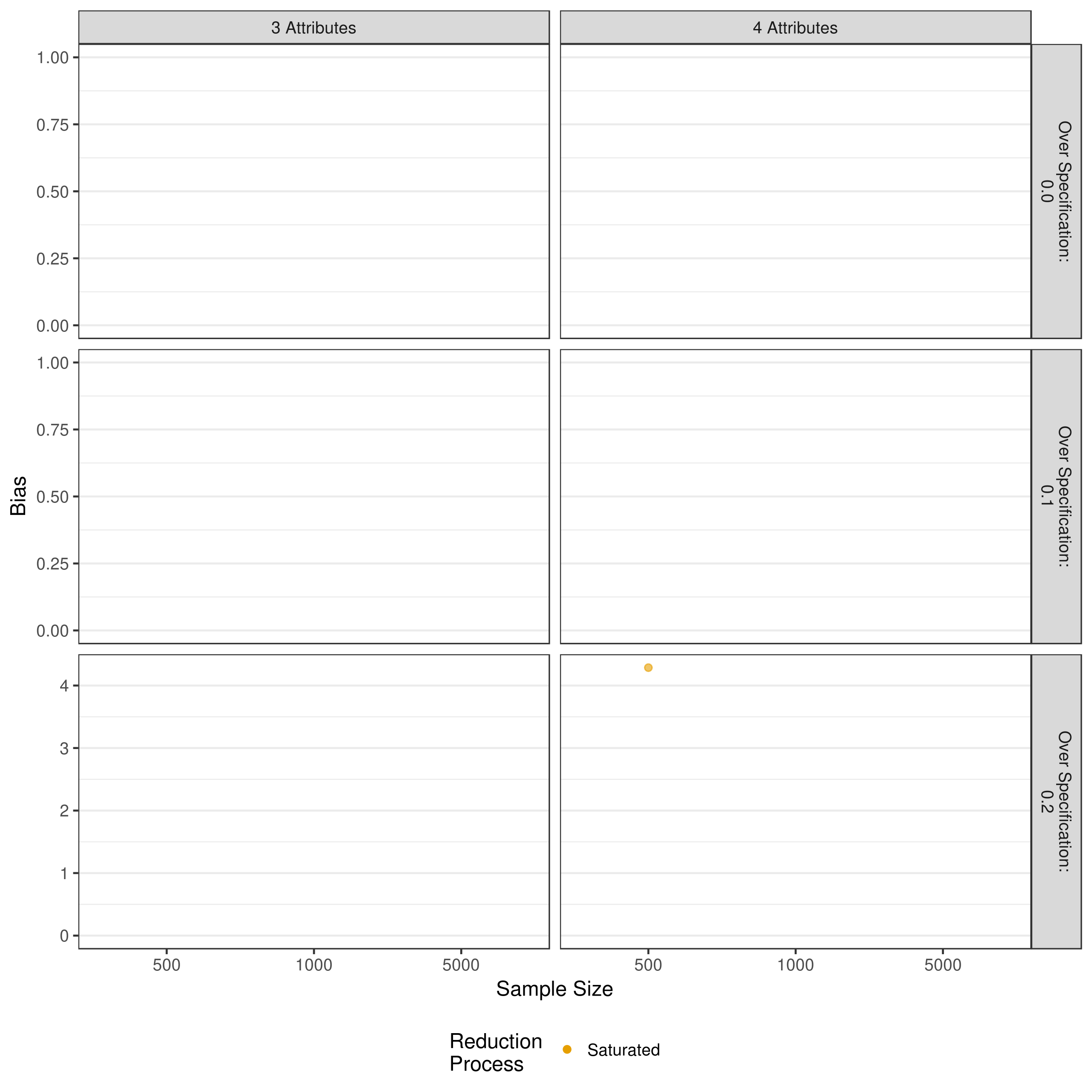Figure A.5: Bias in measurement model 4-way interaction estimates when reducing using p-values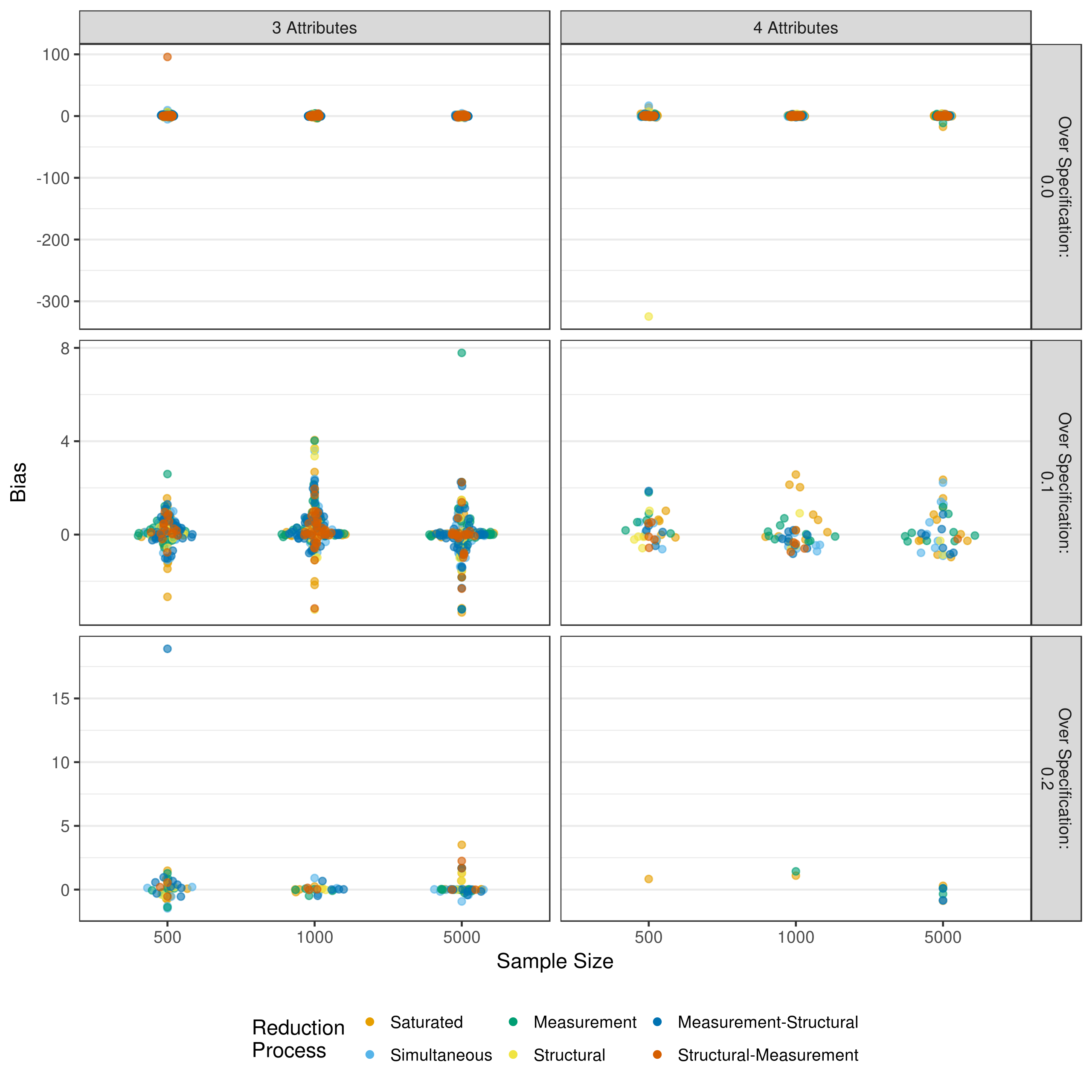Figure A.6: Bias in structural model estimates when reducing using p-values

## A.2 Individual Mean Square Error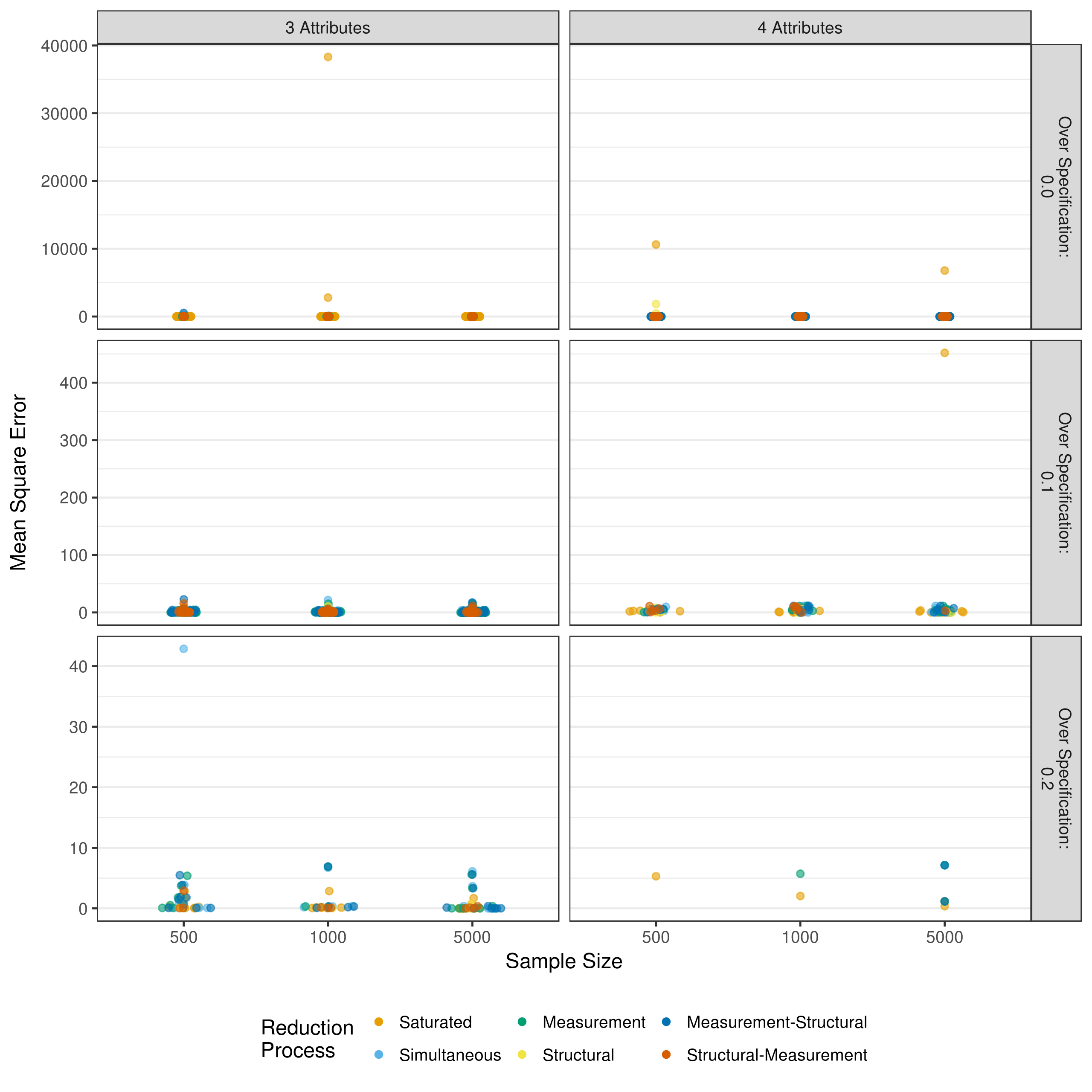Figure A.7: MSE in measurement model intercept estimates when reducing using p-values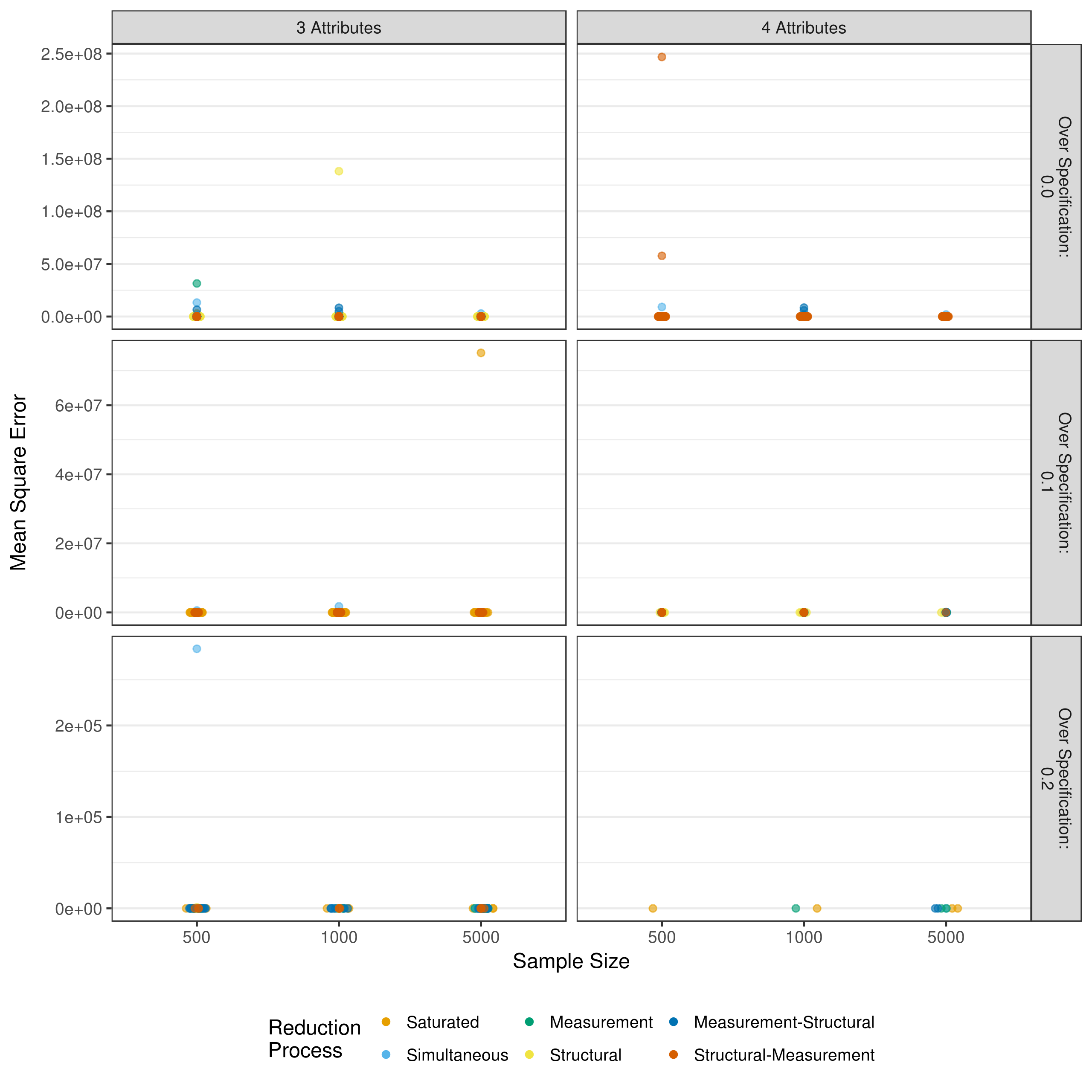Figure A.8: MSE in measurement model main effect estimates when reducing using p-values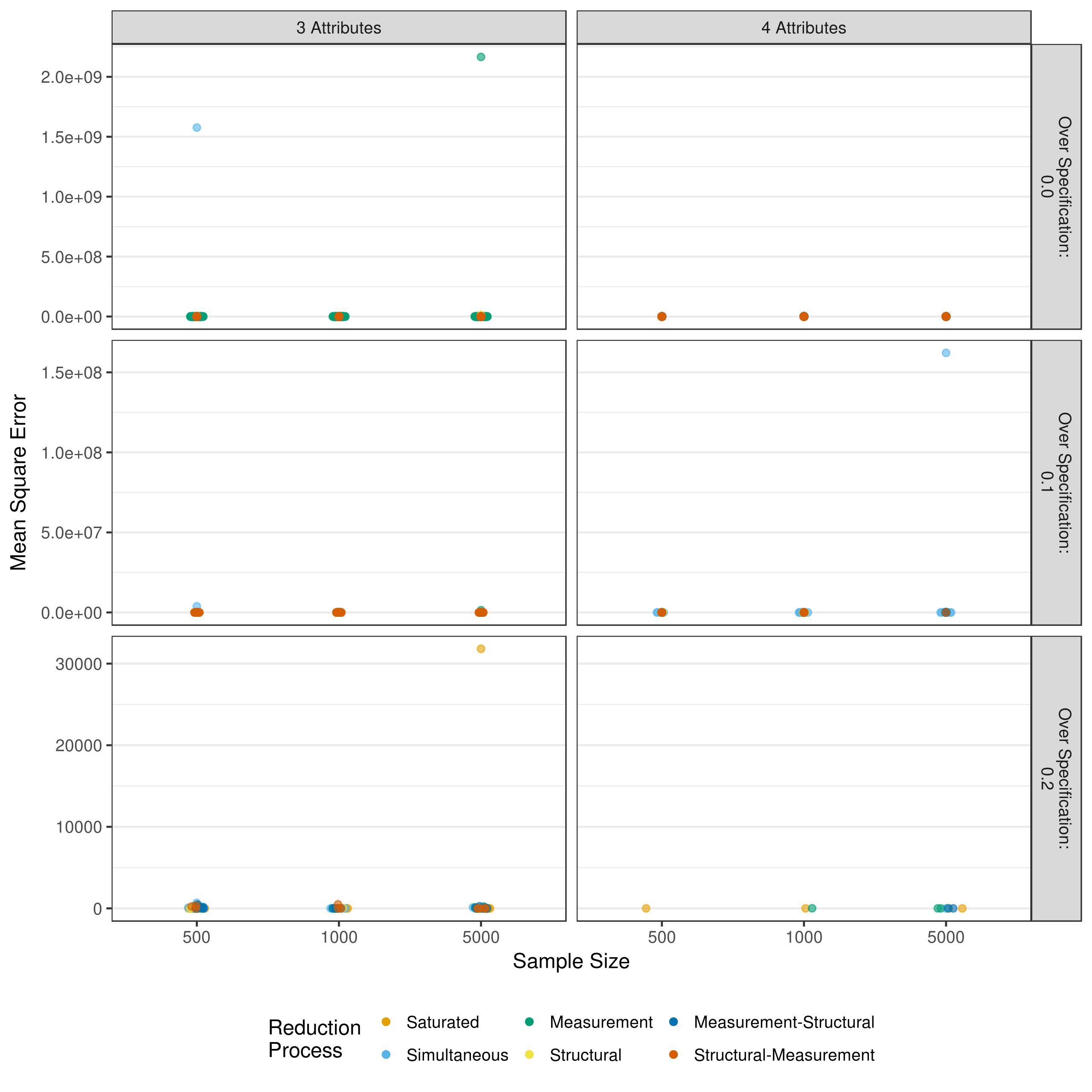Figure A.9: MSE in measurement model 2-way interaction estimates when reducing using p-valuesFigure A.10: MSE in measurement model 3-way interaction estimates when reducing using p-values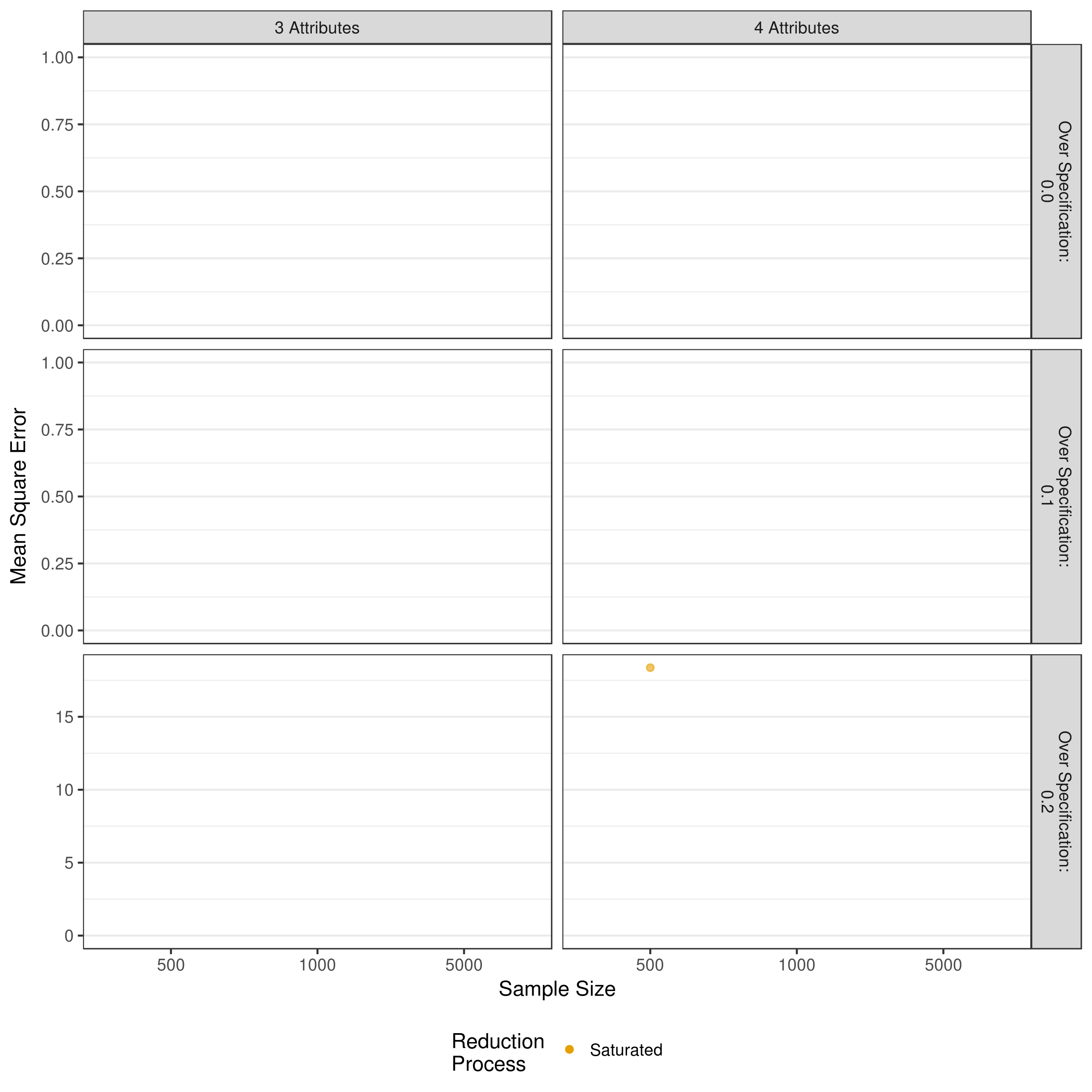Figure A.11: MSE in measurement model 4-way interaction estimates when reducing using p-values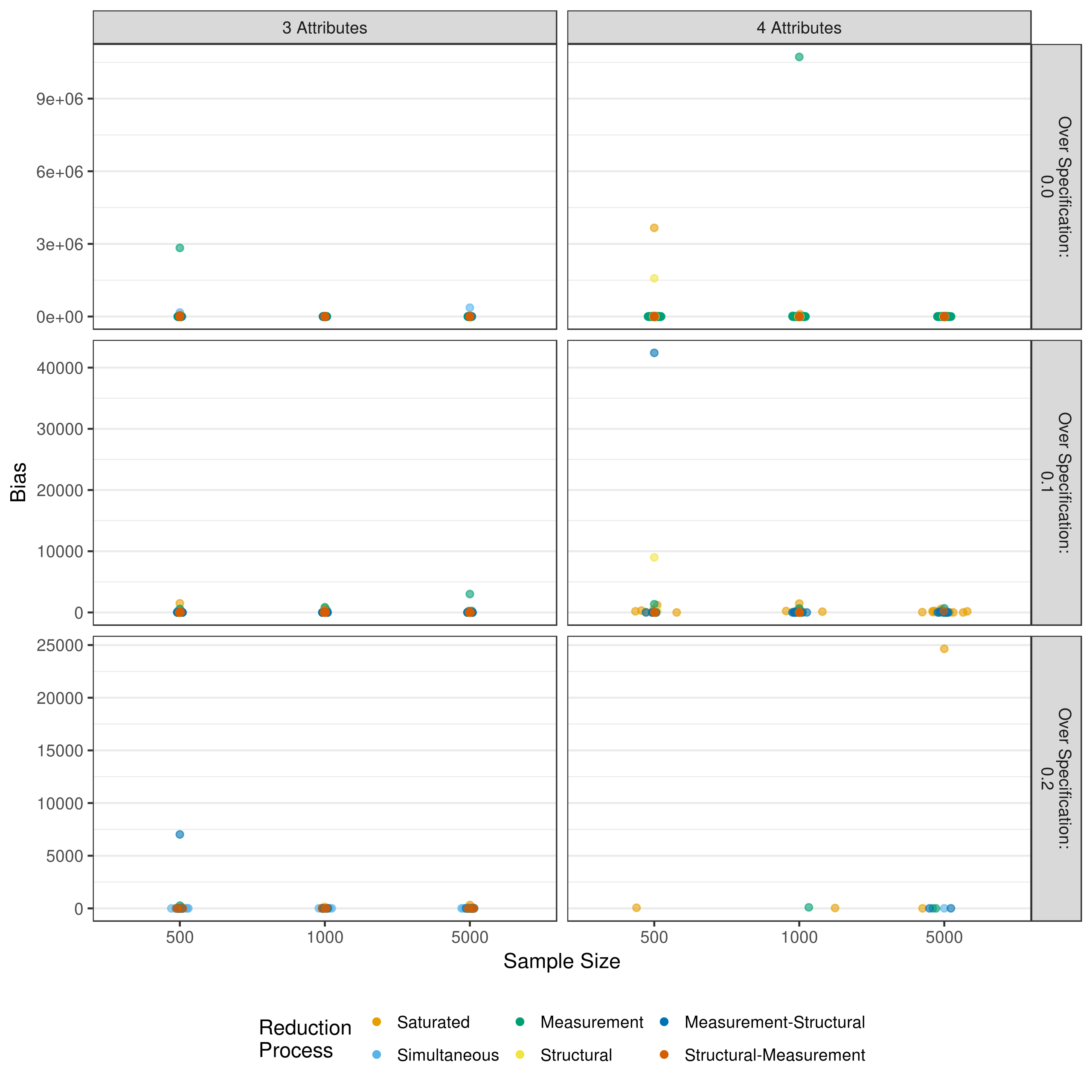Figure A.12: MSE in structural model estimates when reducing using p-values